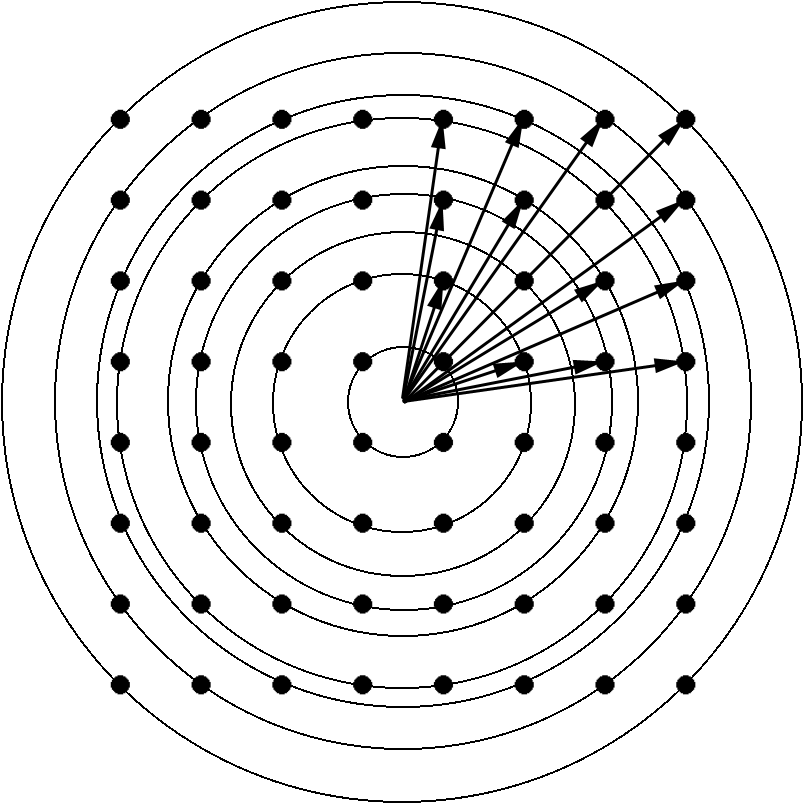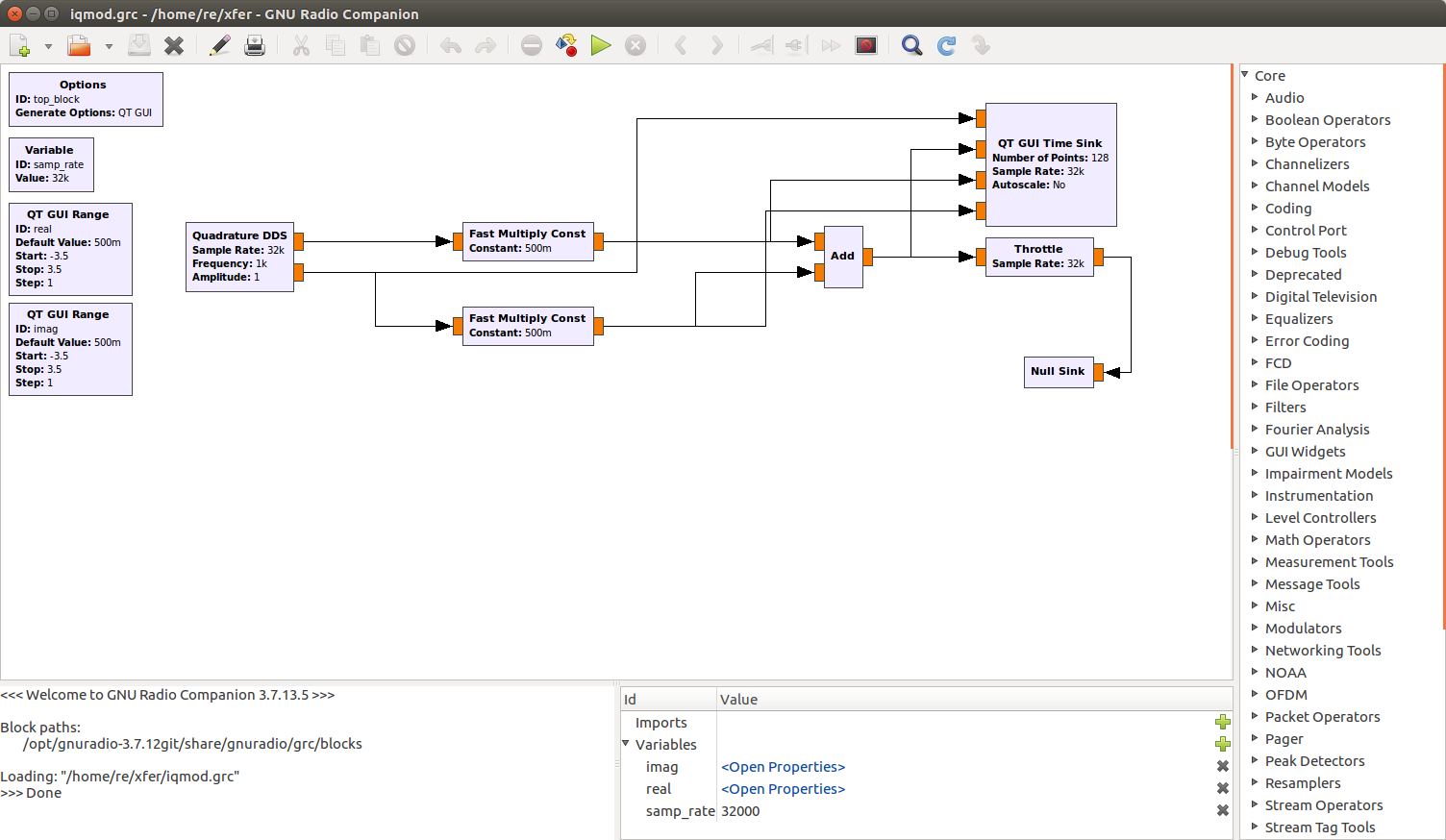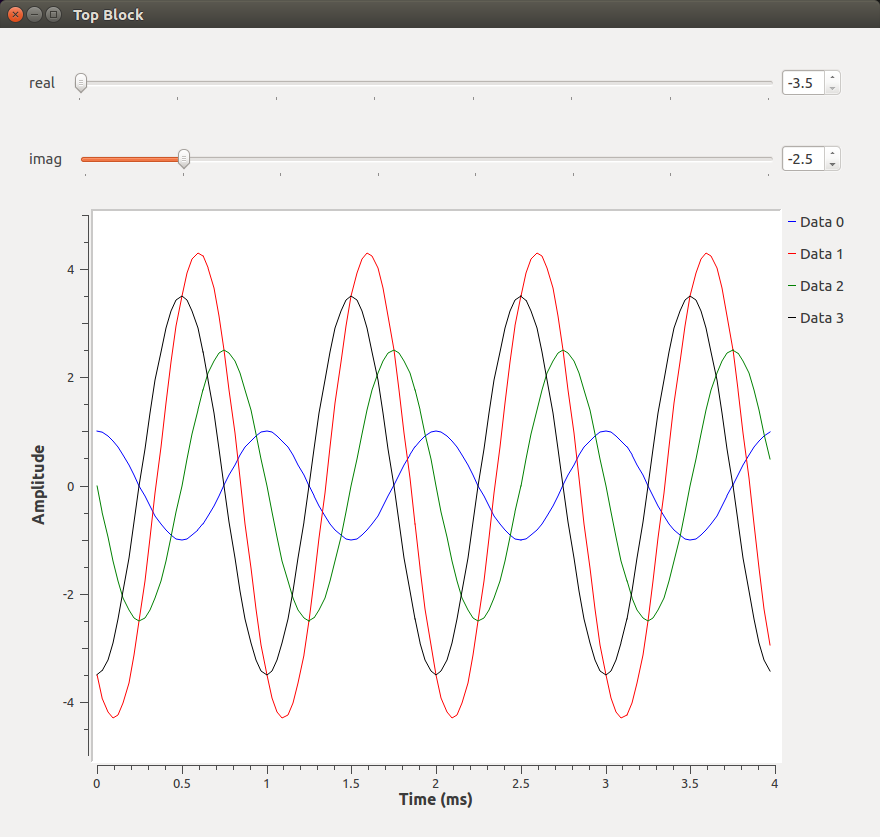# FCC Proposes Authorizing Voluntary All-Digital AM Broadcasting!

Discussion in 'Amplitude Modulation' started by NA5B, Nov 25, 2019.

Tags:For square 64QAM (which is what digital AM uses), there are 9 different amplitude levels and 52 different phase angles. This can be shown graphically.2. The QAM system is generally represented as a square constellation of points in which each point or cell represents a specific Amplitude-phase condition. Every point in the constellation must have a well-defined Amplitude-phase condition at any period in time (Page 5) so the demodulation system can determine how to recreate the bit stream.

https://www.electronics-notes.com/a...ypes-8qam-16qam-32qam-64qam-128qam-256qam.php

My class notes are speaking specifically to a 64-QAM transmitter system and its implementation using a 64-cell/point QAM and layed-out or 'realized' as a "Type-III" constellation, "...the most popular of the three." (Rouphael, Y.,
RF and Digital Signal Processing for Software-Defined Radio: A Multi-Standard Multi-Mode Approach, pp. 61-64, Newnes, 2008).

Page 4 shows the output of each ASK modulator with each modulator producing 8 well-defined states in which each output state contains both a well-defined output voltage and phase. After summation, there are 64 possible Amplitude-phase states.

So your comment, "Not every combination of phase and amplitude may be valid..." seems ambiguous.

Pheel

3. Looks just like how analog modems work.

Its in the audio domain though.

From page 4 of your pdf file.

I Modulator – (5 mV, 0 0 ), (5 mV, 180 0 ), (10 mV, 0 0 ), (10 mv, 180 0 ),
(15 mV, 0 0 ), (15 mV, 180 0 ), (20 mV, 0 0 ) and (20 mV, 180 0 ).
Q modulator - (5 mV, 90 0 ), (5 mV, 270 0 ), (10 mV, 90 0 ), (10 mV, 270 0 ),
(15 mV, 90 0 ), (15 mV, 270 0 ), (20 mV, 90 0 ), and (20 mV, 270 0 ).

Sorry, that's not how it works. Each modulator gets 8 levels. For NRSC-5, it's -3.5, -2.5, -1.5, -0.5, 0.5, 1.5, 2.5, 3.5 (see https://www.nrscstandards.org/stand...s/standards/nrsc-5-d/reference-docs/1012s.pdf page 55 and 56). The amplitude and phase is determined after the summation.

amplitude = sqrt((I*I)+(Q*Q))
phase angle = atan2(I,Q)

If you plug in all of the IQ level combinations to the above equations, you get 9 unique amplitude levels and 52 unique phase angles (as shown in my previous post).

Here's some code to compute the amplitude levels and phase angles.
Code:
```#include <stdio.h>
#include <stdlib.h>
#include <math.h>

int main(int argc, char **argv)
{
int i;
int real_index, imag_index;
double m_64qam_lookup = {-3.5, 3.5, -0.5, 0.5, -2.5, 2.5, -1.5, 1.5};

if (argc != 1) {
fprintf(stderr, "usage: qam64\n");
exit(-1);
}

for (i = 0; i < 64; i++) {
real_index = i & 0x7;
imag_index = i >> 3;
printf("i = %f, q = %f\n", m_64qam_lookup[real_index], m_64qam_lookup[imag_index]);
printf("amplitude = %f\n", sqrt((m_64qam_lookup[real_index] * m_64qam_lookup[real_index]) + (m_64qam_lookup[imag_index] * m_64qam_lookup[imag_index])));
printf("phase = %f\n", atan2(m_64qam_lookup[real_index], m_64qam_lookup[imag_index]) * (180 / M_PI));
}

return 0;
}
```

After taking a shower, I get the authors nomenclature now.

I Modulator – (5 mV, 0 0 ), (5 mV, 180 0 ), (10 mV, 0 0 ), (10 mv, 180 0 ),
(15 mV, 0 0 ), (15 mV, 180 0 ), (20 mV, 0 0 ) and (20 mV, 180 0 ).
Q modulator - (5 mV, 90 0 ), (5 mV, 270 0 ), (10 mV, 90 0 ), (10 mV, 270 0 ),
(15 mV, 90 0 ), (15 mV, 270 0 ), (20 mV, 90 0 ), and (20 mV, 270 0 ).

He's using a phase angle to represent the sign of the level. That is super duper confusing.

Also, raw (no FEC) bit rate is equal to symbol rate * modulation order (6 for 64QAM). Bandwidth is symbol rate * excess bandwidth of the Raised Cosine filter. For example, cable TV 64QAM is 5.056941 Msyms/s * 1.18 excess bandwidth = 5.96719038 or 6 MHz and the bit rate is 5.056941 Msyms/s * 6 * 0.888888... (FEC rate) = 26.97035 Mbps.

7. Maybe for you but for students just learning the theory the (voltage, phase) representation makes sense to them and works just fine for understanding basic 64-QAM. We're not designing for a complete NRSC MA3 AM system. And TV QAM is introduced down the road as another application of QAM.

Yes, in the handout page 3 each ASK modulator gets 8 bits or levels.

The NRSC reference docs show one suggested implementation/methodology. As I said in the handout, there are many ways to implement a 64-QAM system.

And yes I am familiar with that document and it is geared toward meeting the specifications for designing a complete system with all the bells and whistles.

12.2.1 Primary Subcarriers The 256x25 primary interleaver matrices (PU and PL) are mapped to 64-QAM constellations. The elements of these matrices are six-bit words. To map each six-bit word within an interleaver matrix to a subcarrier location within the vector X, the following steps are taken: 1. Read a six-bit word from an element within an interleaver matrix. 2. Map the six-bit word from Step 1 to a complex constellation value using Table 12-1. 3. Scale the I and Q components of the complex constellation value from Step 2 using the appropriate amplitude scale factor from Table 12-11. 4. Map the scaled constellation value from Step 3 to the appropriate element of X using Table 12-2 or Table 12-3.

If you are doing this in software that's fine but we're not. We're implementing this simple modulator in hardware so the students can use their knowledge of combinatorial logic to implement the functions.

And again, we're not designing a complete NRSC MA3 AM system to feed a BE AM-6A.

Pheel

Last edited: Dec 11, 2019
8. Good observation.

In fact telephone modems used 4 and 8-PSK, 16-QAM, 64-QAM, and Trellis-coded QAM.

You can think of this all-digital AM system as a more complex modem modulating a carrier.

Pheel

I had never considered the intermediate signals after the I and Q mixers. Just the inputs and output of the IQ modulator.

The entire IQ modulator can be easily simulated in GNU Radio. The Quadrature DDS block represents the LO with sine and cosine outputs. The Fast Multiply block represents a mixer (a mixer is just a multiplier, that's why the schematic symbol for a mixer is a circle with an X inside). And of course, the Add block represents the summer. The QT Time Sink creates a graphical display like an oscilloscope.The blue trace is the cosine output of the LO (and the reference phase). The red trace is the output of the modulator. The green trace is the Q mixer output (at amplitude 1.5 and 90 degrees) and the black trace is the I mixer output (at amplitude 2.5 and 0 degrees). The modulator output amplitude is sqrt((I+Q)*(I+Q)) = 2.915 and the phase angle is atan2(I,Q) = 59.036 degrees.Then setting the real input value to -3.5 causes the I mixer output to be 3.5 amplitude and 180 degrees and setting the imaginary input to -2.5 causes the Q mixer output to be 2.5 amplitude and 270 degrees. The modulator output amplitude is sqrt((I+Q)*(I+Q)) = 4.301 and the phase angle is atan2(I,Q) = -125.537 degrees.10. Consider it any way you like. 64-QAM modulation can be implemented in many different ways.

A later, more advanced class introduces students to digital systems simulators and software.

Students have to learn how to implement the physical hardware before they advance to system simulation.

As far as systems simulators, there are many different analog/digital/hybrid systems simulator software packages out there that can accomplish the same thing.

Once they graduate, the company they work for will train them as to how to use their specific system's simulator.

Pheel

Last edited: Dec 13, 2019